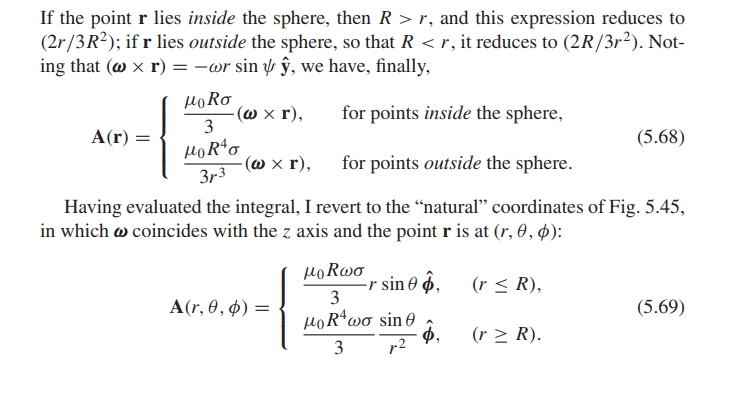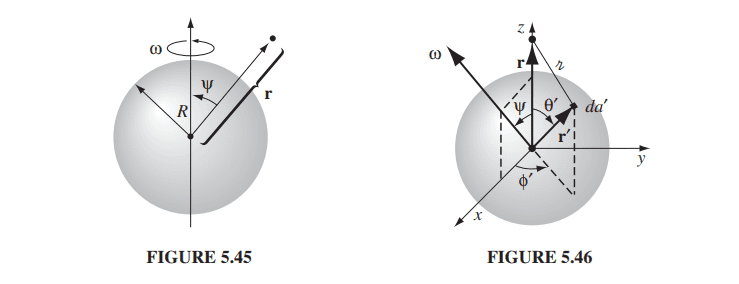# Vector potential in spherical coordinatesin this problem i can solve v = ω x r = <0, -ωrsinψ, 0> in cartesian coordinates

but i don't understand A in sphericle coordinates why?

(inside) A = ⅓μ0Rσ(ω x r) = ⅓μ0Rσωrsin(θ) θ^

how to convert coordinate ?